Previous: 3.3.5.2 Follow-Each-Recoil Method Up: 3.3.5 Material Damage - Next: 3.3.5.4 Amorphization

### 3.3.5.3 Point Defect Recombination

Besides the energy loss mechanism of mobile particles and the damage generation process, thermal effects have to be considered when simulating ion implantation. All atoms in a solid perform random motions which result in diffusion effects of impurities and point defects. Thereby interstitials and vacancies can approach each other close enough to recombine to a regular lattice atom.

Several approaches have been proposed to model this recombination effect either in combination with the Kinchin-Pease damage model or in combination with the Follow-Each-Recoil method.

An empirical model which can be combined with the Kinchin-Pease model is given in . The recombination process is subdivided into recombination within a single cascade and recombination with point defects from previous cascades. The number of point defects surviving recombination within a single cascade is determined by an ion species dependent factor(recombination factor) which is multiplied with the number of generated point defect pairs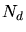calculated by the modified Kinchin-Pease model (3.143). The recombination with previously generated point defects is considered by a recombination probability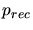. The expected value of Frenkel pairs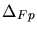remaining after recombination per generated Frenkel pair can be calculated by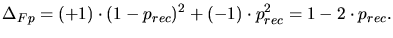(3.149)

The first term takes into account that the vacancy and the interstitial do not recombine which results in an additional Frenkel pair. The probability of this process is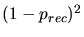. The second terms considers a full recombination process with a probability of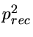, which reduces the number of Frenkel pairs by one.is assumed to be proportional to the local interstitial and vacancy concentration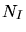,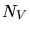and to a species dependent saturation concentration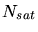.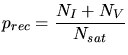(3.150)

Combining all contributions the total number of new stable point defects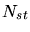generated by a primary recoil can therefore be calculated by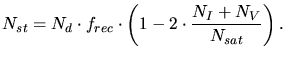(3.151)

This expression is more general than the one proposed in  where the vacancy and interstitial concentration are assumed to be equal. Tab. 3.5 summarizes experimentally determined values ofand(, , , ). A similar model was proposed by Posselt in . The models mainly differ in placing the interstitials as will be explained in Sec. 3.3.5.

Table 3.5: Parameters for the empirical recombination model for different ion species.

 Ion speciesBoron 0.125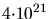cm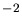Phosphorus 1.000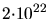cmArsenic 2.000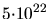cmThere are several approaches to model the point defect recombination process if a Follow-Each-Recoil method is applied for the calculation of the point defects (, , , ). All these methods are based in principle on the same concepts which will be summarized in the following by looking in detail at the damage generation processes.

1. When a mobile particle transfers more energy than the displacement energy to a stable atom in a crystalline solid a recoil is generated. If this recoil is removed from a lattice position a vacancy is left behind. If an interstitial atom was hit the number of interstitials is reduced by one.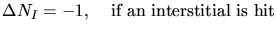(3.152)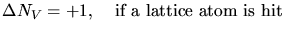(3.153)andare the number of interstitials and vacancies,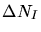andindicate the change in the number of interstitials and vacancies, respectively.
2. If a vacancy has been generated it can recombine with an interstitial.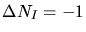(3.154)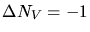(3.155)

There are two approaches to model the recombination probability. By the first approach () it is assumed that the recombination probability is a linear function of the interstitial concentration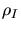.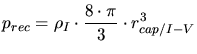(3.156)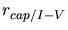is a capturing radius for interstitial-vacancy pairs.

By the alternative approach () it is also suggested to distinguish between the recombination of point defects originating from the same cascade and point defects originating from different cascades. While the recombination of point defects originating from different cascades is assumed to be linearly proportional to the interstitial concentration, the recombination of point defects originating from the same cascade is assumed to be constant (independent of the interstitial concentration). In order quantify this assumption a constant value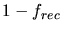is added for the calculation of the recombination probability.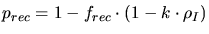(3.157)is the recombination probability within a single cascade and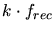is the proportionality coefficient for inter-cascade recombination.
3. Instead of recombining with an interstitial a vacancy can recombine with an impurity atom which is located at an interstitial site.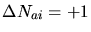(3.158)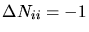(3.159)(3.160)(3.161)is a capturing radius for impurity-vacancy pairs. A different capturing radius is required for each impurity species because their thermal mobilities are different.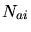is the number of activated (located at lattice positions) impurities,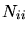and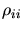are the number and the concentration of interstitial impurities.
4. When a mobile particle comes to rest an interstitial atom is generated.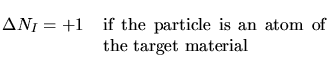(3.162)(3.163)

5. Such an interstitial atom can recombine with a previously generated vacancy.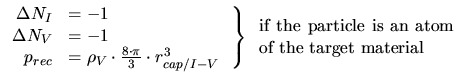(3.164)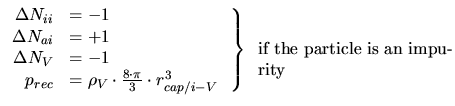(3.165)

6. Besides recombination the point defects can form clusters as indicated by several molecular dynamic simulations (, ) and isolated point defects can recombine with these clusters. The reaction radius of a cluster which determines the clustering probability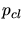is assumed to be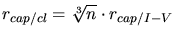().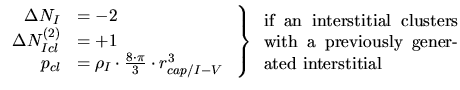(3.166)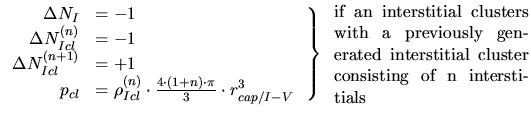(3.167)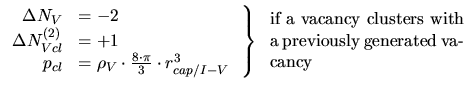(3.168)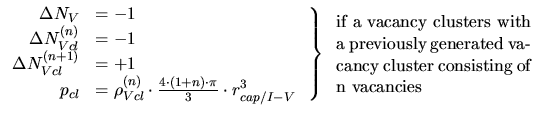(3.169)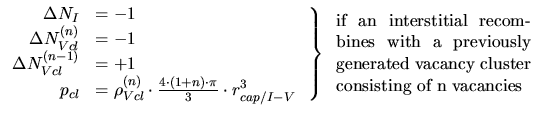(3.170)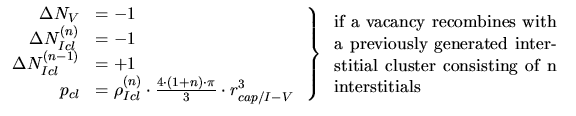(3.171)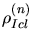,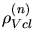,,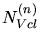are the densities and numbers of interstitial and vacancy clusters consisting of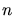point defects.

Applying these mechanisms the generation of several defect species like isolated interstitials and vacancies, impurity-interstitial pairs and point defect and impurity-point defect clusters can be modeled. But at least isolated interstitials and vacancies have to be considered in the simulation to correctly handle the de-channelling mechanism. Therefore at least the mechanisms mentioned in 1., 2. and 4. have to be applied during the simulation, while all other mechanisms just provide additional information for the output of the Monte-Carlo ion implantation simulation.

A. Hoessiger: Simulation of Ion Implantation for ULSI Technology